# Interval Notation

Interval Notation

In this mini-lesson, we will explore the world of interval notation.

We will try answering questions like what is meant by interval notation, what are the different types of intervals, what are notations for different types of intervals, how to convert inequality to interval notation, and discover other interesting aspects of it.

You can check out the interactive simulations to know more about the lesson and try your hand at solving a few interesting practice questions at the end of the page.

Before we get started, let's have a look at the story shown below.

Ms. Dolma is just showing the weather forecast for the upcoming few days.

She says that there is going to be a heavy snowstorm with at least 4 but a maximum of 9 inches of snow.Can you guess the amount of snow we could expect based on this information?

She described this information in an interval.

We don't want you to worry, and get your snow shower ready as we, at Cuemath, are here to help you understand the interval notation in a clear way.

You can represent an interval using inequalities, an interval notation, or even on a number line.

In this short lesson, we will learn the way of expressing an inequality using the interval notation and graph them on a number line.

## Lesson Plan

 1 What Is Meant by Interval Notation? 2 Important Notes on Interval Notation 3 Solved Examples on Interval Notation 4 Interactive Questions on Interval Notation

## What Is Meant By Interval Notation?

Interval Notation is a way of expressing a subset of real numbers by the numbers that bound them.

We can use this notation to represent inequalities.

### Examples of Interval Notation

Suppose we want to express the set of real numbers $$\{x|-2<x<5\}$$ using an interval.

This can be expressed as interval notation $$(-2,5)$$.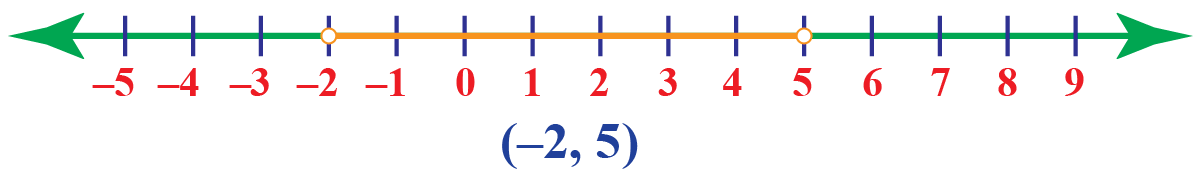The set of real numbers can be expressed as $$(-\infty,\infty)$$.## What Are the Different Types Of Intervals?

There are three types of intervals.

• Open Interval
• Closed Interval
• Half-Open Interval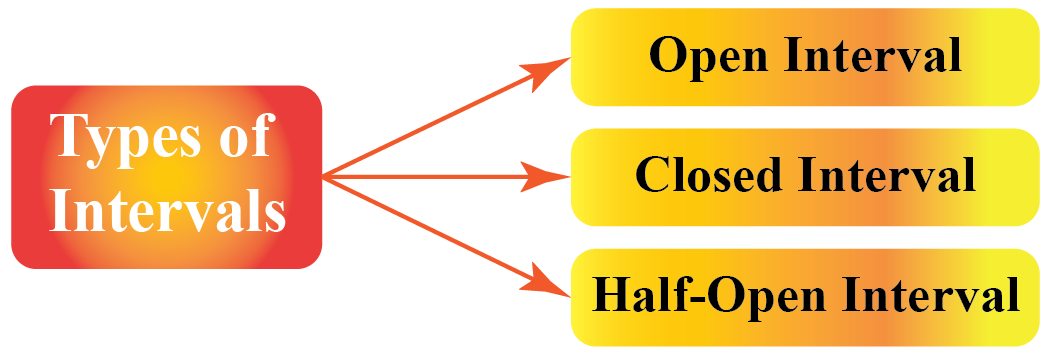### Open Interval

This type of interval does not include the endpoints of the inequality.

For example, the set $$\{x|-3<x<1\}$$ does not include the endpoints, -3 and 1

This is expressed using open interval notation: $$(-3,1)$$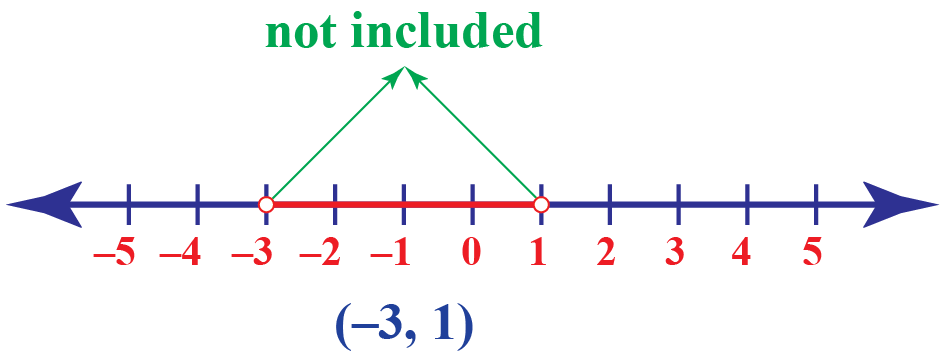### Closed Interval

This type of interval includes the endpoints of the inequality.

For example, the set $$\{x|-3 \leq x \leq 1\}$$ include the endpoints, -3 and 1

This is expressed using closed interval notation: $$[-3,1]$$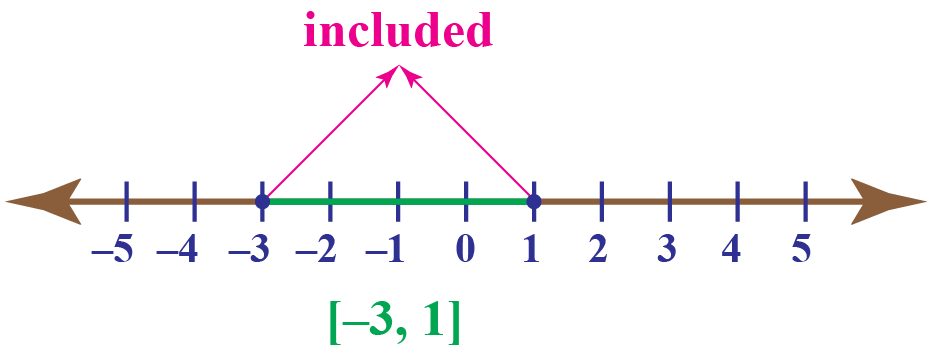### Half-Open Interval

This type of interval includes only one of the endpoints of the inequality.

For example, the set $$\{x|-3 \leq x < 1\}$$ include the endpoint -3

This is expressed using half-open interval notation: $$[-3,1)$$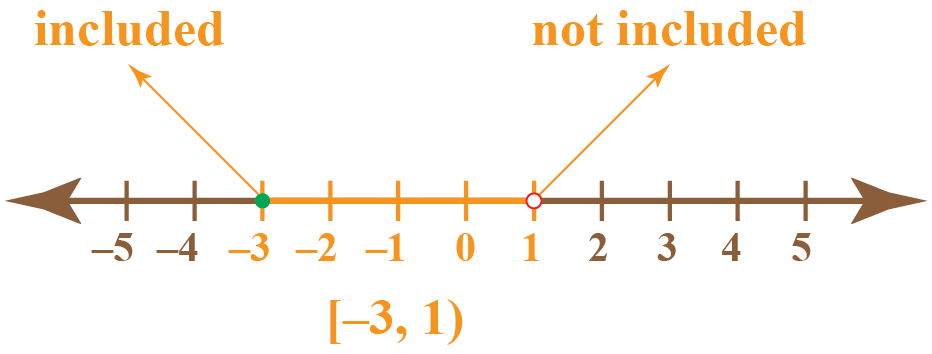## What Are Notations For Different Types of Intervals?

### The Symbol for Interval Notation

The notations we use for different intervals are:

• $$[\;\;]$$: This is a square bracket that is used when both the endpoints are included in the set.
• $$[\;\;]$$: This is a round bracket that is used when both the endpoints are excluded in the set.
• $$(\;\;]$$: This is a semi-open bracket that is used when the left endpoint is excluded and the right endpoint is included in the set.
• $$[\;\;)$$: This is also a semi-open bracket that is used when the left endpoint is included and the right endpoint is excluded in the set.

## Number Line Representation of Different Types Of Intervals

Look at the handy table that distinguishes between all the types of intervals.

Interval Notation Inequality Number Line Type of Interval
$$(a,b)$$ $$\{x|a< x < a\}$$Open Interval
$$[a,b]$$ $$\{x|a \leq x \leq a\}$$Closed interval
$$[a,\infty)$$ $$\{x|x \geq a\}$$Half-Open Interval
$$(a,\infty)$$ $$\{x|x > a\}$$Half-Open Interval
$$(-\infty, a)$$ $$\{x|x < a\}$$Half-Open Interval
$$(-\infty, a]$$ $$\{x|x \leq a\}$$Half-Open Interval

### Graph of Interval Notation using Number Line

Here is a solver for interval notation.

Drag the slider to change the interval notation and then click on the "New Problem" button to experiment with different intervals.

Hope you enjoyed the graph of interval notation.

## How To Convert Inequality To Interval Notation?

Follow the steps mentioned below to convert an inequality to interval notation.

1. Graph the solution set of the interval on a number line.
2. Write the numbers in the interval notation with a smaller number appearing first on the number line on the left.
3. If the set is unbounded on the left, use the symbol $$-\infty$$ and if it is unbounded on right, use the symbol $$\infty$$.

Let's take a few examples of inequality and convert them to interval notation.

Inequality Number Line Interval Notation
$$x \leq 3$$$$(-\infty, 3]$$
$$x < 5$$$$(-\infty, 5)$$
$$x \geq 2$$$$(2,\infty]$$

## Solved Examples

 Example 1

For a person to be elected as the president, he should be a minimum of 35 years old.Give an interval representing this information.

### Solution

Let's represent the age of the president by $$A$$.

It is given that the person's age $$A$$ should be a minimum of 35

This means that $$A$$ should be either greater than or equal to 35

Hence, this is represented by the inequality, $$A \geq 10$$

 So, the required interval is $$[10, \infty)$$.
 Example 2

For a school to participate in an Olympiad exam, the number of students from the school should be a minimum of 10Represent this using an interval notation.

Solution

Let $$x$$ represent the number of students participating in a class, then $$x$$ should be greater than or equal to 10

This means $$x\geq 10$$

The interval notation is $$(10, \infty)$$.

 So, the number of students is represented by $$(10, \infty)$$.
 Example 3

Lola needs at least 1500 calories a day but the calorie intake should not exceed 1800 calories.Represent the possible amount of calories she could eat using interval notation.

Solution

Let's represent the number of calories by $$x$$.

The inequality representing the possible amount of calories is given by $$1500 \leq x \leq 1800$$

The interval notation for this inequality is $$[1500, 1800]$$

 The required interval notation is $$[1500, 1800]$$.Challenging Questions
 1. Can you determine the subset of real numbers represented by the number line shown below? Express this using the interval notation.## Interactive Questions

Here are a few activities for you to practice.

## Let's Summarize

The mini-lesson targeted the fascinating concept of Interval Notation. The math journey around Interval Notation starts with what a student already knows, and goes on to creatively crafting a fresh concept in the young minds. Done in a way that not only it is relatable and easy to grasp, but also will stay with them forever. Here lies the magic with Cuemath.

At Cuemath, our team of math experts is dedicated to making learning fun for our favorite readers, the students!

Through an interactive and engaging learning-teaching-learning approach, the teachers explore all angles of a topic.

Be it worksheets, online classes, doubt sessions, or any other form of relation, it’s the logical thinking and smart learning approach that we, at Cuemath, believe in.

## 1. What is interval notation on a graph?

When we represent the solution set of an interval on a number line, that is a graph for the interval notation.

## 2. What is the $$\cup$$ symbol for interval notation?

$$\cup$$ symbol is used to denote the union of two or more intervals.

## 3. How do you exclude numbers in interval notation?

We use the round brackets to exclude numbers in interval notation.

More Important Topics
Numbers
Algebra
Geometry
Measurement
Money
Data
Trigonometry
Calculus